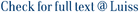We study the problem of computing hierarchical drawings of layered graphs when some pairs of edges are not allowed to cross. We show that deciding the existence of a drawing satisfying at least k non-crossing constraints from a given set is NP-hard, even if the graph is 2-layered and even when the permutation of the vertices on one side of the bipartition is fixed. We then propose simple constant-ratio approximation algorithms for the optimization version of the problem, which requires to find a maximum realizable subset of constraints, and we discuss how to extend the well-known hierarchical approach for creating layered drawings of directed graphs so as to minimize the number of edge crossings while maximizing the number of satisfied constraints. © 2005 Elsevier B.V. All rights reserved.

Crossing-constrained hierarchical drawings / Finocchi, Irene. - In: JOURNAL OF DISCRETE ALGORITHMS. - ISSN 1570-8667. - 4:2(2006), pp. 299-312. [10.1016/j.jda.2005.06.001]

### Crossing-constrained hierarchical drawings

#### Abstract

We study the problem of computing hierarchical drawings of layered graphs when some pairs of edges are not allowed to cross. We show that deciding the existence of a drawing satisfying at least k non-crossing constraints from a given set is NP-hard, even if the graph is 2-layered and even when the permutation of the vertices on one side of the bipartition is fixed. We then propose simple constant-ratio approximation algorithms for the optimization version of the problem, which requires to find a maximum realizable subset of constraints, and we discuss how to extend the well-known hierarchical approach for creating layered drawings of directed graphs so as to minimize the number of edge crossings while maximizing the number of satisfied constraints. © 2005 Elsevier B.V. All rights reserved.
##### Scheda breve Scheda completa Scheda completa (DC)2006
crossing minimization; graph drawing; hierarchical layout; np-completeness
Crossing-constrained hierarchical drawings / Finocchi, Irene. - In: JOURNAL OF DISCRETE ALGORITHMS. - ISSN 1570-8667. - 4:2(2006), pp. 299-312. [10.1016/j.jda.2005.06.001]
File in questo prodotto:
File
finocchiJDA.pdf

Solo gestori archivio

Tipologia: Versione dell'editore
Licenza: DRM (Digital rights management) non definiti
Dimensione 216.07 kB
Utilizza questo identificativo per citare o creare un link a questo documento: `https://hdl.handle.net/11385/192641`
•2
•1